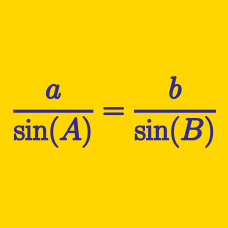Geometry

Solving Triangles: Level 3 Challenges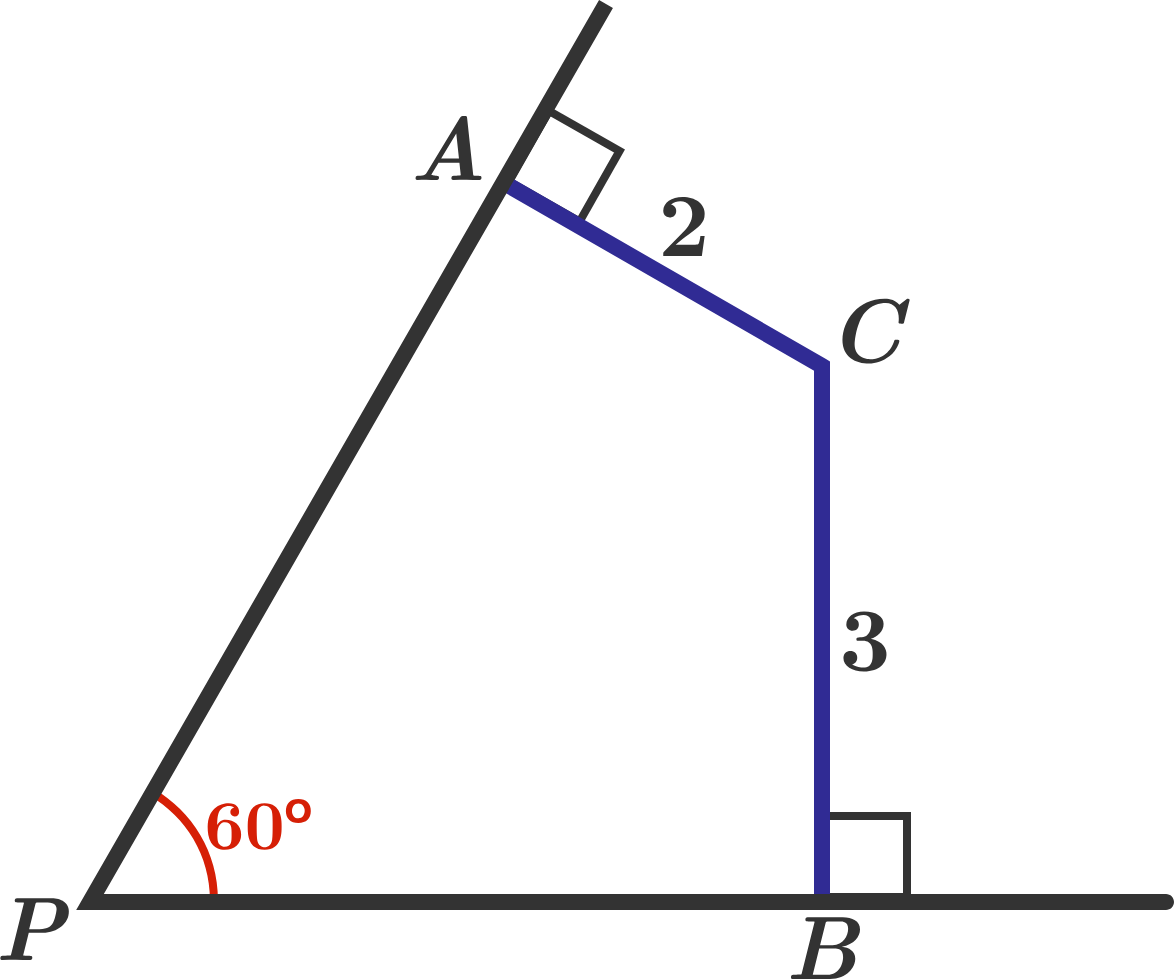Find $PC.$

Note: This length can be expressed as $\sqrt{\dfrac{A}{B}}$, where $A,B$ are coprime positive integers. Submit the value of $A+B$ as your answer.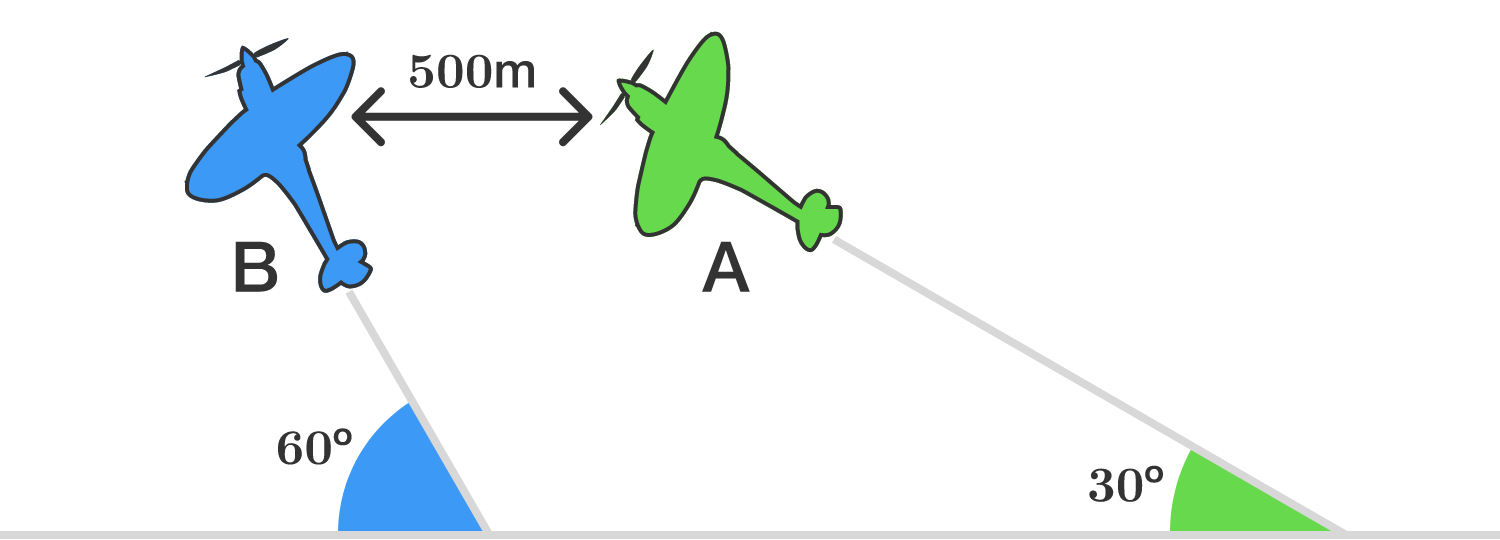Aeroplanes A and B are flying with constant speed in the same vertical plane at angles 30° and 60° with respect to the horizontal, respectively, as shown in the figure.

The airspeed of A is $100 \sqrt{3}$ meters per second. Initially, an observer in A sees B directly ahead and at the same altitude at a (horizontal) distance of 500 m.

Assuming they take no evasive action and collide, after how many seconds does this happen?

An ant is lost in a square, and his distances to the vertices of the square are 7, 35, 49, and $x.$ Find $x.$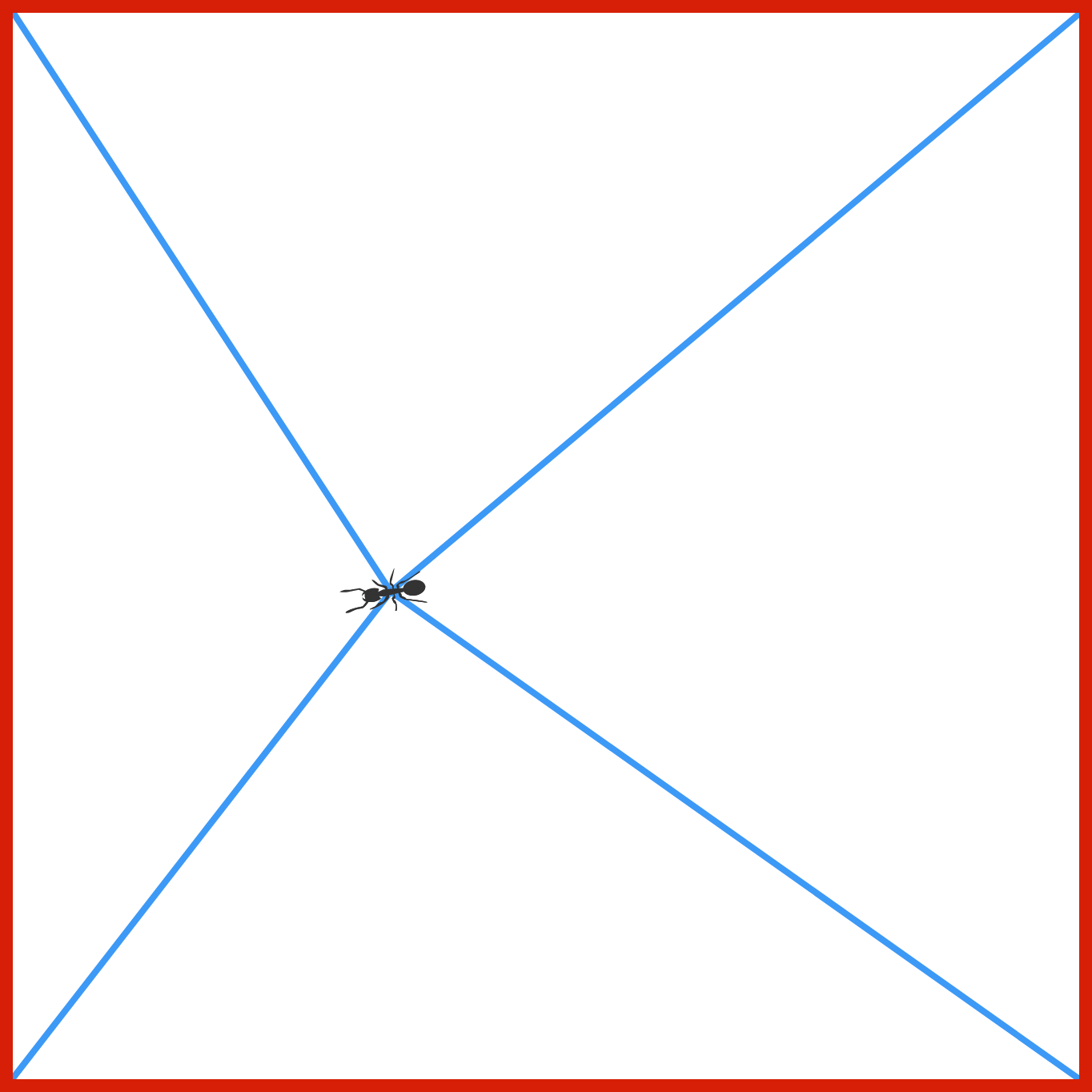Note: The image is not drawn to scale.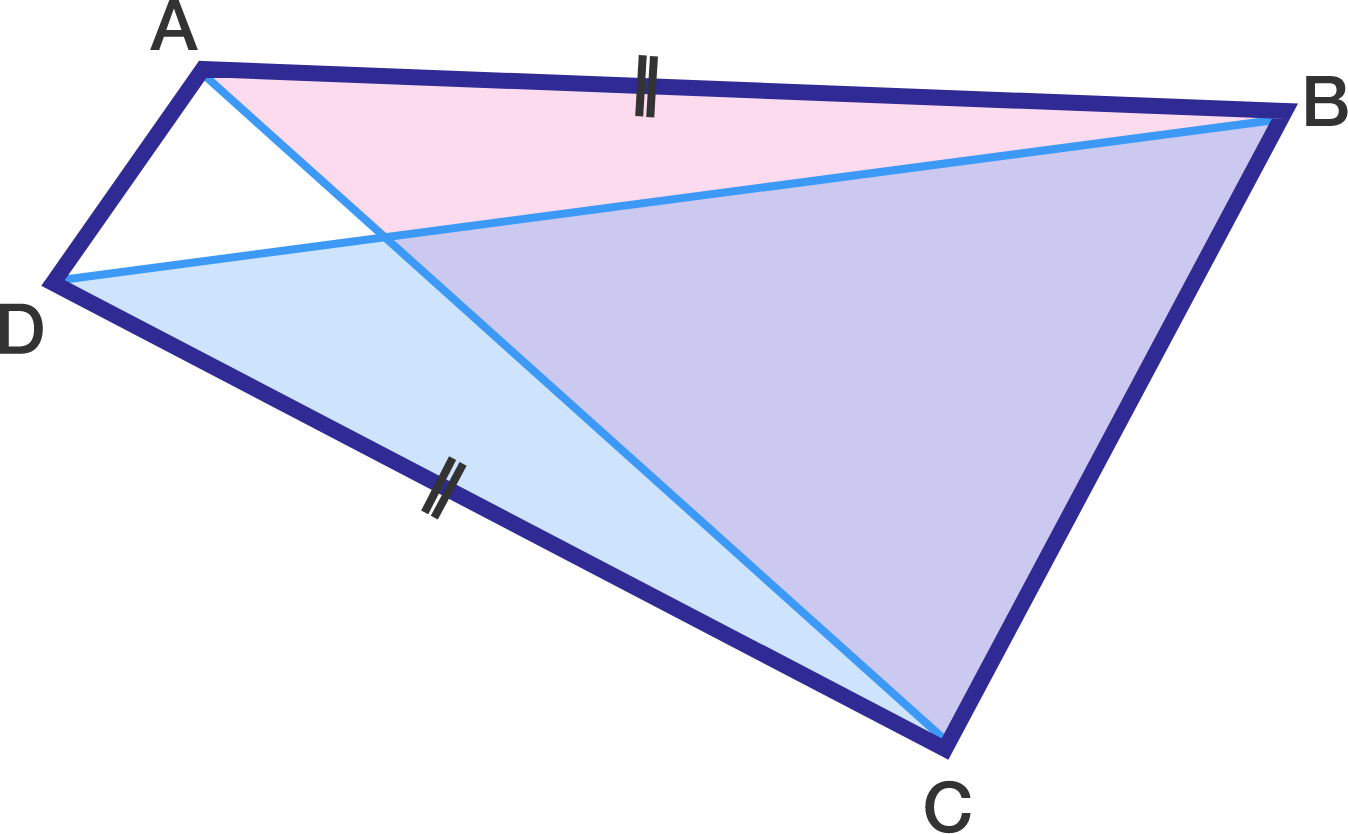Quadrilateral $ABCD$ has $AB=CD,$ and the acute angles $B$ and $C$ satisfy $\sin B = \frac{4}{9}$ and $\sin C= \frac{5}{6}.$ Find $\frac{\text{area of }\color{#D61F06}{\triangle ABC}}{\text{area of }\color{#3D99F6}{\triangle BDC}}.$

• The image is not drawn to scale.

In triangle $ABC$, $\angle ACB = 90^\circ$. Points $A, D, E,$ and $B$ are consecutive points on side $AB$ such that $\overline{AD}=\overline{DE}=\overline{EB}$. If there exists $\theta$ such that $\overline{CD} = 5 \cos \theta$ and $\overline{CE} = 5 \sin \theta$, what is $\overline{AB}^2?$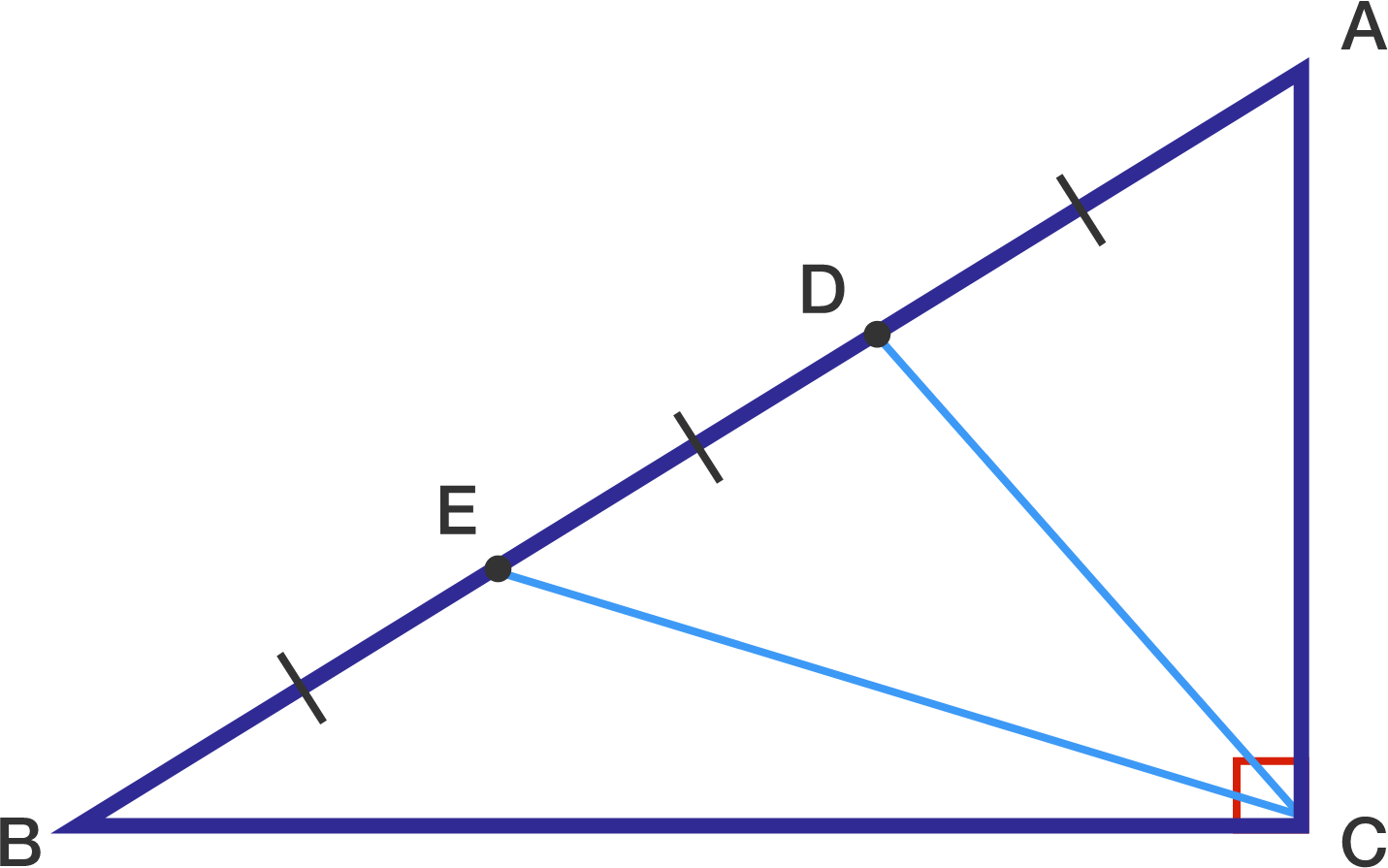×